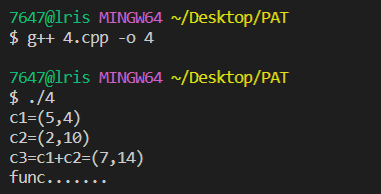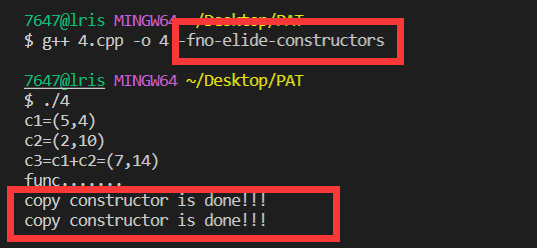# c++中有些重载运算符为什么要返回引用

• 核心主旨：事实上运算符返回void、返回对象本身、返回对象的引用都是可以的，并不是说一定要返回引用，只不过这三者的含义不同。但是有一点，运算符的出现要保证能够连续的运算。

## 返回void

``````void operator+(classA &a,classA &b)
{
//重载函数的定义
}

int main()
{
classA a,b,c,d;
a=b+c+d;
}``````

## 返回类的对象

​ 那上面的例子中，如果你想让`a=b+c+d`可以正确运行，那重载函数的返回值得是类的对象或者对象的引用。所以我们重载运算符返回值为类的对象是为了实现连续的运算。

​ 那如果返回对象，代码的结果是啥样呢？这里我们用“+”运算符做例子：

``````#include<iostream>
using namespace std;

class Complex
{
public:
Complex(double r=0.0,double i=0.0):real(r),imag(i){}
Complex(const Complex &cc)
{
this->real = cc.real;
this->imag = cc.imag;
cout<<"copy constructor is done!!!"<<endl;
}

Complex operator+(Complex &c1);         //这里我们返回的是类的对象
friend ostream &operator<<(ostream &out,const Complex &c);    //这个函数先不管，只是为了输出复数类重载一下"<<"运算符

private:
double real;
double imag;
};

Complex Complex::operator+(Complex &c1)
{
this->real+=c1.real;
this->imag+=c1.imag;
return *this;               //这里是返回了类的对象哦，所以是要调用拷贝构造的
}

ostream &operator<<(ostream &out,const Complex &c)
{
out<<"("<<c.real<<","<<c.imag<<")";
return out;
}

int main()
{
Complex c1(5,4),c2(2,10),c3;
cout<<"c1="<<c1<<endl;
cout<<"c2="<<c2<<endl;
c3=c1+c2;                   //主要看这一句
cout<<"c3=c1+c2="<<c3<<endl;

return 0;
}``````

``````c1=(5,4)
c2=(2,10)
copy constructor is done!!!
c3=c1+c2=(7,14)``````

​ 以上就是一个复数类重载”+“运算符的例子，当我们返回对象的时候，就可以做一个连续的运算，而不会像返回void类型一样使得表达式无法进行（当然啦，还是再说一下，语法没有问题，就是没啥结果）。

​ 那么可以看到我们重载”+“运算符函数的定义最后是`return *this`，即一个新的对象,是会去调用拷贝构造的。

## 返回类的对象的引用

​ 既然返回类的对象已经可以满足重载后运算符的连续运算这一性质，那为啥有的重载运算符要给引用呢，不多此一举咩？

​ 那这里先给大家补个引用的知识，C++中引用的作用（部分作用）：

• 是一个变量的别名，且不分配内存

• 是一种绑定的关系，永久性婚姻，即声明的时候必须初始化，一经声明，不可更改

• 可以对引用再次引用，多次引用后，某一变量具有多个别名

这里解释第三点：

``````int a;
int &b=a;
int &c=b;

​ 那么以上的特性，体现了引用是一个提高效率的东西，那么在重载运算符中，如果返回引用，那么将不会去调用拷贝构造，甚至是析构。看如下代码：

``````#include<iostream>
using namespace std;

class Complex
{
public:
Complex(double r=0.0,double i=0.0):real(r),imag(i){}
Complex(const Complex &cc)
{
this->real = cc.real;
this->imag = cc.imag;
cout<<"copy constructor is done!!!"<<endl;
}
Complex &operator+(Complex &c1);            //这里我们返回的是类的对象的引用
friend ostream &operator<<(ostream &out,const Complex &c);    //这个函数先不管，只是为了输出复数类重载一下"<<"运算符

private:
double real;
double imag;
};

Complex & Complex::operator+(Complex &c1)
{
this->real+=c1.real;
this->imag+=c1.imag;
return *this;               //这里是返回了类的对象的引用哦，不用调构造了，因为是一个已经存在的对象，不是新的对象
}

ostream &operator<<(ostream &out,const Complex &c)
{
out<<"("<<c.real<<","<<c.imag<<")";
return out;
}

int main()
{
Complex c1(5,4),c2(2,10),c3;
cout<<"c1="<<c1<<endl;
cout<<"c2="<<c2<<endl;
c3=c1+c2;                   //主要看这一句
cout<<"c3=c1+c2="<<c3<<endl;

return 0;
}``````

``````c1=(5,4)
c2=(2,10)
c3=c1+c2=(7,14)``````

​ 如果返回对象的引用，那么就不会调用拷贝构造函数，当然啦也不会调用析构，因为返回的*this是一个旧的对象，不是新的，所以这样效率就上去了。

## 运算符重载函数——成员函数类内定义版本

``````class Complex
{
public:
Complex(double r=0.0,double i=0.0):real(r),imag(i){}
Complex(const Complex &cc)
{
this->real = cc.real;
this->imag = cc.imag;
cout<<"copy constructor is done!!!"<<endl;
}
Complex operator+(Complex &c1)
{
this->real+=c1.real;
this->imag+=c1.imag;
return *this;
}
private:
double real;
double imag;
};
``````

## 运算符重载函数——成员函数类外定义版本

``````class Complex
{
public:
Complex(double r=0.0,double i=0.0):real(r),imag(i){}
Complex(const Complex &cc)
{
this->real = cc.real;
this->imag = cc.imag;
cout<<"copy constructor is done!!!"<<endl;
}
Complex operator+(Complex &c1);
private:
double real;
double imag;
};

Complex Complex::operator+(Complex &c1)
{
this->real+=c1.real;
this->imag+=c1.imag;
return *this;
}``````

## 运算符重载函数——友元函数版本

``````class Complex
{
public:
Complex(double r=0.0,double i=0.0):real(r),imag(i){}
Complex(const Complex &cc)
{
this->real = cc.real;
this->imag = cc.imag;
cout<<"copy constructor is done!!!"<<endl;
}
friend Complex operator+(Complex &c1,Complex &c2);
private:
double real;
double imag;
};

Complex operator+(Complex &c1,Complex &c2)
{
c1.real+=c2.real;
c1.imag+=c2.imag;
return c1;
}``````

# 拷贝构造函数

​ 这里我们进一步研究一下拷贝构造函数。众所周知，拷贝构造就是复制一个一模一样的东西，它的三个应用场景：

• 1、当我调用的函数形参是一个对象，就需要

• 2、当我创建类的对象的时候，用另一个类的对象去给它赋值，就需要

• 3、如果函数的返回值是类的对象，函数执行完成返回调用者时。

那请看下面的例子，还是刚才的例子，我们在main函数加了点东西，还加了一个func函数：

``````#include<iostream>
using namespace std;

class Complex
{
public:
Complex(double r=0.0,double i=0.0):real(r),imag(i){}
Complex(const Complex &cc)
{
this->real = cc.real;
this->imag = cc.imag;
cout<<"copy constructor is done!!!"<<endl;
}
Complex &operator+(Complex &c1);            //这里我们返回的是类的对象
//Complex operator+(Complex &c1);           //这里我们返回的是类的对象
friend ostream &operator<<(ostream &out,const Complex &c);    //这个函数先不管，只是为了输出复数类重载一下"<<"运算符

private:
double real;
double imag;
};

Complex & Complex::operator+(Complex &c1)
{
this->real+=c1.real;
this->imag+=c1.imag;
return *this;               //这里是返回了类的对象哦，所以是要调用拷贝构造的
}

ostream &operator<<(ostream &out,const Complex &c)
{
out<<"("<<c.real<<","<<c.imag<<")";
return out;
}

Complex func()          //新加的函数
{
Complex temp(10,20);
cout<<"func......."<<endl;
return temp;                //返回类的对象
}

int main()
{
Complex c1(5,4),c2(2,10),c3;
cout<<"c1="<<c1<<endl;
cout<<"c2="<<c2<<endl;
c3=c1+c2;                   //主要看这一句
cout<<"c3=c1+c2="<<c3<<endl;

//以下两句话是等价的，新加的
Complex c4=func();
//Complex c4(func());

return 0;
}``````​ 大家发现问题了嘛，为啥只有一个func的输出，`Complex c4(func函数返回的对象)`应该会调用拷贝构造函数啊，也就是创建c4这个对象的时候，得调用其拷贝构造函数，而且func函数`return temp`应该也会调用拷贝构造啊，结果一次都没调用，为啥没有调用呢？

​ 答案是：RVO（return value optimization），也就是G++对返回值进行了优化，看下图：​ 懂了嘛，添加这个选项，两个拷贝构造的调用都出来了，所以有的时候没有输出你想要的，可能是编译器优化掉了，并不是这个过程没有发生。那这里`-fno-elide-constructors`表示将C11的RVO优化关闭。那关于返回值引用的具体内容，可以我总结的《返回值优化》。

## 小插曲

​ 不知道大家有没有一个疑惑，为啥拷贝构造函数的形参的是一个对象的引用：

``````class Complex
{
Complex(const Complex &cc){};
}``````

​ 这个是一个标准的拷贝构造函数对吧，形参是一个引用，那为啥不能是一个对象呢，例如下面这个代码：

``````class Complex
{
Complex(Complex cc){};
}``````

​ 那为啥不能写成这样呢？我给大家拆解一下：

``````class Complex
{
//如果你这么写，当c2(c1)这句执行后，会有Complex cc=c1，那此时岂不是又要调用拷贝构造函数对cc进行初
//始化？那就陷入一个死循环状态，可以理解吧
Complex(Complex cc){};

//那如果你加了引用，为啥不会出现这样的死循环
//因为const Complex &cc=c1，这句话并不会再调用拷贝构造函数，这是把c1和引用cc绑定在一起，这两是一
//个东西，他不是初始化懂吧，而Complex cc=c1这句话是一个初始化的语句，那你对一个对象进行初始化，给的
//参数还是另一个对象，那你不得调用拷贝构造嘛，是吧。
Complex(const Complex &cc){};
}

int main()
{
Complex c1;
Complex c2(c1);         //这句话啥意思，不就是用c1来为c2做初始化么，也就是要调用构造喽
}
``````

``````class Complex
{

Complex(Complex cc){};

//首先你要知道在这个例子中，哪里会调用拷贝构造
//main函数的Complex c2(c1);会调用拷贝构造，即用c1来初始化c2，
//那么对应到函数的参数就是  const Complex &cc=c1，对吧
//如果没有const，那么c1是一个右值，cc是一个左值，右值是不能赋给左值的
//但加了const就可以，const （type） &是一个万能引用，既可以被右值赋值，也可以被左值赋值
//所以一般来说，只要形参不在函数内修改其内容，我们一般都是给const &的，例如运算符重载函数、拷贝构造、移动构造
Complex(const Complex &cc){};
}

int main()
{
Complex c1;
Complex c2(c1);
}``````

lionの金库